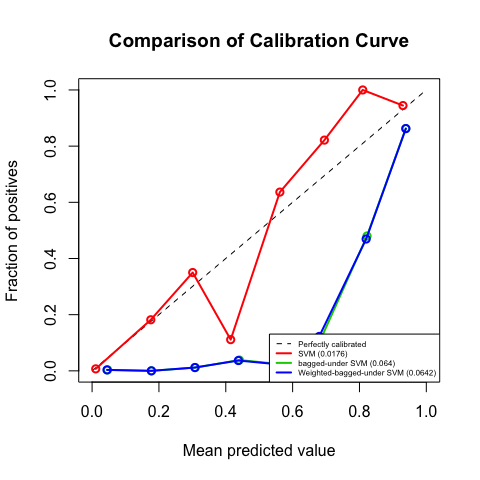# Getting started with imbCalib

#### 2020-05-03

When doing classification, we not only want the prediction results, but also the corresponding probabilty of the decision. The ideal probability shall give us a sense of confidence about the predicted labels. For example, given a sample, if the probability for the prediction is 80%, then approximately 80% samples with the same features actually have the predicted label. For applications where we need to use confidence to support decision making, it is vital to have good estimates of probabilities.

But unlike logistic regression, where the prediction is based on probabilities, many supervised learning methods don’t come natually with probabilities. For example, Support Vector Machine is performed based on margins, instead of probabilities. The probabilities given by SVM model is actually calibrated using Platt’s scaling. Another commonly used calibration method is Isotonic regression. Check Niculescu-Mizil and Caruana 2005 for more details.

One big issue of these methods is that they only work for balanced data, and when it comes to the imbalanced data case, they usually underestimate the probabilities for the minority class instances. To solve this problem, Wallace and Dahabreh 2014 proposed using bagged undersampled method to calibrate probabilities. And this is the methodology basis of this package.

## Imbalanced Dataset

Class imbalance happens when the number of instances in each class is not equal. And in the imbalanced scenario, the rare events are usually misclassified. Imbalance would affect not only classfication results, but also probability calibration.

A synthesized imbalanced dataset imbalance is provided in this package and it can be loaded with data(imbalance). In this dataset, about 5% are in the positive class. There are 8 covariates and 1 binary response. The positive label is 1.

data(imbalance)
names(imbalance)
>  "X1" "X2" "X3" "X4" "X5" "X6" "X7" "X8" "y"
mean(imbalance$y) >  0.055 ## Metrics ### Why AUC is not enough? In the evaluation of model performances, accuracy is not enough for classification problems, and AUC can provide us more information. But AUC itself is not enough to evaluate the goodness of probability calibration. The following example illustrates that the scale of the probabilities would not affect AUC. Two sets of probabilities can have the same AUC, while they show different levels of confidence. Therefore, AUC is not appropriate to evaluate the goodness of probability calibration. # simulate data threshold <- 0.6 set.seed(99) y.true <- (runif(10) > threshold) * 1 set.seed(92) y.prob <- runif(10) y.pred <- (y.prob > threshold) * 1 # print out the simulated data y.true >  0 0 1 1 0 1 1 0 0 0 y.prob >  0.06959910 0.64357977 0.30941810 0.64467185 0.82390023 0.83391847 >  0.11737230 0.47910214 0.04046598 0.18796384 y.pred >  0 1 0 1 1 1 0 0 0 0 # auc in the original scale auc(roc(y.true, y.prob, direction = "<", quiet = TRUE)) > Area under the curve: 0.6667 # auc using the rank of the probabilities auc(roc(y.true, rank(y.prob), direction = "<", quiet = TRUE)) > Area under the curve: 0.6667 # auc using the probability-like rank auc(roc(y.true, rank(y.prob) / 50, direction = "<", quiet = TRUE)) > Area under the curve: 0.6667 # plot kernel density plot(density(y.prob), col = 'red', main = 'Kernel Density Curve', xlab = 'Probability Value', xlim = c(-0.5, 1.5), ylim = c(0, 5.5), lty = 1) lines(density(rank(y.prob) / 50), col = 'blue', lty = 1) legend('topright', legend = c("Original Probability", "Rescaled Probability"), col = c('red', 'blue'), lty = c(1, 1))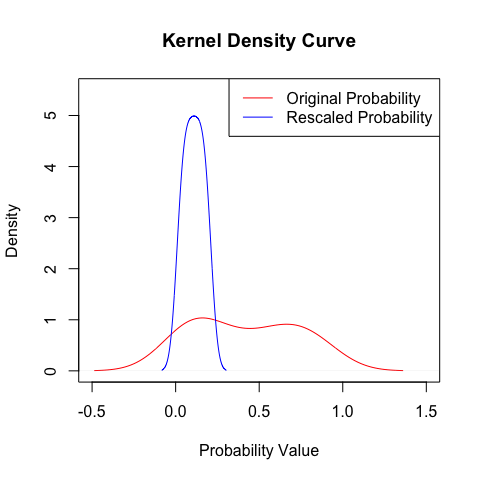### Brier Score Brier score measures the fit of probability estimates to the observed data. It is defined as the mean squared difference between the observed labels and the estimated probability. A smaller value means a better calibration. $BS = \frac{\sum_{i=1}^N (y_i - \hat{P}(y_i | x_i))^2}{N}$ To obtain the standard Brier score, run brier function. The first argument should be the true labels, and the second argument should be the calibrated probabilities. y.prob <- c(0.45454545, 0.36363636, 0.63636364, 0.18181818, 0.45454545, 0.09090909, 0.27272727, 0.81818182, 0.63636364, 0.63636364) y.true <- c(0, 0, 1, 1, 0, 1, 1, 0, 0, 0) brier(y.true, y.prob) >  0.4181818 ### Stratified Brier Score Stratified Brier Score was proposed by Wallace and Dahabreh 2014 to evaluate the goodness of calibration under the imbalanced scenario. Unlike the standard Brier score, which only considers the overall matching, it takes care of both the minority and the majority class. It consists of two parts: Brier score for the positive class, and Brier score for the negative class. They are defined as follows. $BS^+ = \frac{\sum_{y_i=\text{pos_label}} (y_i - \hat{P}(y_i | x_i))^2}{N_{pos}}$ $BS^- = \frac{\sum_{y_i=\text{neg_label}} (y_i - \hat{P}(y_i | x_i))^2}{N_{pos}}$ stratifiedBrier function would output a list with three elements: the overall Brier score, the positive Brier socre, as well as the negative Brier score. stratifiedBrier(y.true, y.prob) >$BS
>  0.4181818
>
> $BS+ >  0.5392562 > >$BS-
>  0.3374656

## Calibration Curve

Visualization usually helps us better understand the problem of the model more quickly and intuitively. In this package, calibCurve shows two plots: the top one is the calibration curve along with a perfectly calibrated dashed line, and the bottom one is the histogram of the calibrated probabilities. The corresponding Brier score is shown in the legend. comparisonPlot plots the calibration curves from several models or from several calibration methods.

We now use the dataset imbalance to see how the two plotting functions work. In the following, we consider four models: Logistic Regression, Naive Bayes Classifier, Random Forest Classifier, and Support Vector Machine(SVM). For simplity, the default parameters are used. For each model, we plot their individual calibration curve respectively, and then draw a comparison plot.

# load the dataset and split into train and test
data(imbalance)
set.seed(123)
split <- sample.split(imbalance$y, SplitRatio = 0.75) train_set <- subset(imbalance, split == TRUE) test_set <- subset(imbalance, split == FALSE) X.test <- subset(test_set, select = -y) y.test <- subset(test_set, select = y)[,1] # Logistic Regression lr <- glm(y ~ ., data = train_set, family = "binomial") prob.lr <- predict(lr, X.test, type = "response") calibCurve(y.test, prob.lr, "Logistic")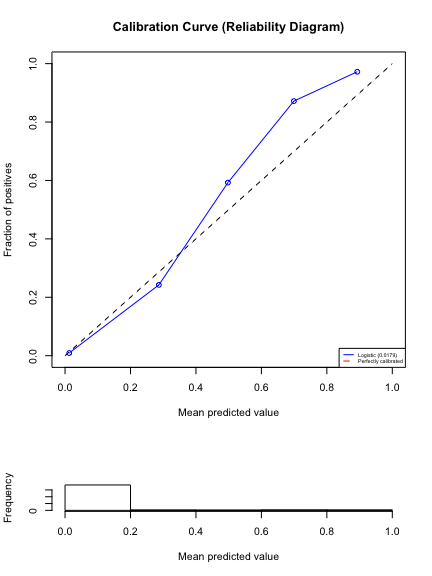# Naive Bayes nb <- naiveBayes(y ~ ., data = train_set) prob.nb <- as.data.frame(predict(nb, X.test, type = "raw"))$1
calibCurve(y.test, prob.nb, "Naive Bayes")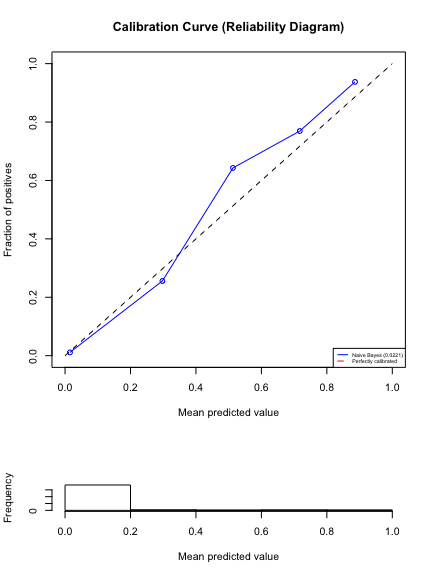# Random Forest Classifier
rfc <- randomForest(as.factor(y) ~ ., data = train_set)
prob.rfc <- as.data.frame(predict(rfc, X.test, type = "prob"))$1 calibCurve(y.test, prob.rfc, "Random Forest")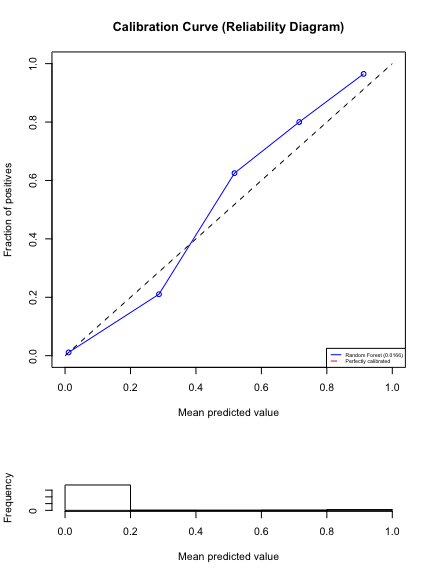# Support Vector Machine Classifier svc <- svm(formula = as.factor(y) ~ ., data = train_set, type = 'C-classification', kernel = 'linear', probability = TRUE) pred <- predict(svc, X.test, probability = TRUE) prob.svc <- as.data.frame(attr(pred, "probabilities"))$1
calibCurve(y.test, prob.svc, "SVM")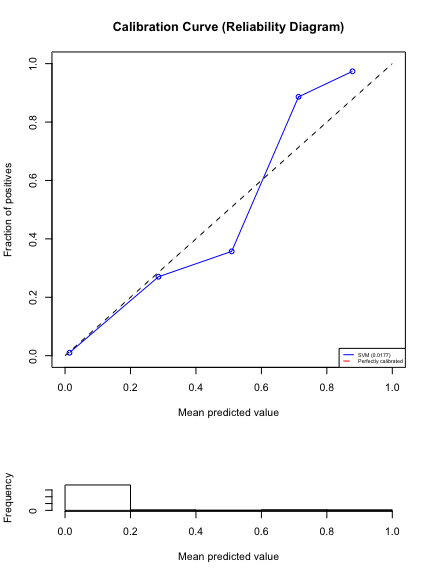comparisonPlot(y.test, list(prob.lr, prob.nb, prob.rfc, prob.svc),
c("Logistic Regression", "Naive Bayes", "Random Forest", "SVM"))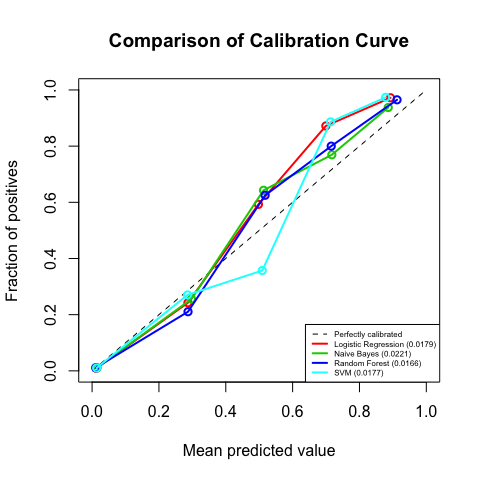## Bagged Undersampled Calibration

bagCalibrate uses the bagged undersampled method to calibrate the probabilities for imbalanced datasets. There are two versions of bagging combination: the weighted average and the simple average, as defined below. To choose which version to use, specify the ntimes argument. When ntimes = 1, it is the simple average. And when ntimes > 1, it is the weighted average, and the weight is obtained using ntimes runs on each sampled dataset.

$\hat{P}(y_i | x_i) = \frac{1}{k}\sum_{j=1}^k \hat{P}_j(y_i | f_{ij})$ $\hat{P}(y_i | x_i) = \frac{1}{z}\sum_{j=1}^k \frac{1}{\text{Var}(\hat{P}_j(y_i | f_{ij}))} \hat{P}_j(y_i | f_{ij}),$ where $z = \sum_{j=1}^k \frac{1}{\text{Var}(\hat{P}_j(y_i | f_{ij}))}$

Models are trained using trainset, and predictions are made on newX. response_name specifies the name of the response in the trainset, and model specifies the model to work on. The function can now work with logistic regression models 'lr', naive Bayes models 'nb', random forest classifiers 'rf', and support vector machine classifiers 'svm'. nbags specifies how many samples sets are used for bagging. Note that a large value will not lead to overfitting and will reduce the variance more, with the only cost being heavy computation load. And to speed up the bagging procedure, parallel computing is enabled. Find the number of cores in your computer, and then set ncluster.

# show the arguments of bagCalibrate
args(bagCalibrate)
> function (trainset, newX, response_name, model, formula = as.factor(y) ~
>     ., pos_label = 1, nbags = 25, ntimes = 1, ncluster = 4, ...)
> NULL

# find the number of cores in your computer
library(doParallel)
detectCores()
>  4

Here, we use SVM as an example, and compare the standard calibration method with the bagged undersampled method. Note that the same formula of SVM is used for both methods. As the stratified Brier scores suggest, the bagged undersampled method can greatly mitigate the effect of imbalance in the positive class calibration, without much sacrifice in the negative class and the overall.

# standard probability calibration
svc <- svm(formula = as.factor(y) ~ ., data = train_set,
type = 'C-classification', kernel = 'linear', probability = TRUE)
pred <- predict(svc, X.test, probability = TRUE)
prob.svc <- as.data.frame(attr(pred, "probabilities"))$1 stratifiedBrier(y.test, prob.svc) >$BS
>  0.01763159
>
> $BS+ >  0.2391364 > >$BS-
>  0.004739772
# simple version of bagged undersampled calibration
bag.prob.svm <- bagCalibrate(train_set, X.test, 'y', model='svm',
type = 'C-classification', kernel = 'linear',
nbags = 30, ntimes = 1, ncluster = 4)
stratifiedBrier(y.test, bag.prob.svm)
> $BS >  0.06395149 > >$BS+
>  0.06681419
>
> $BS- >  0.06378488 # weighted version of bagged undersampled calibration weighted.bag.prob.svm <- bagCalibrate(train_set, X.test, 'y', model='svm', type = 'C-classification', kernel = 'linear', nbags = 30, ntimes = 20, ncluster = 4) stratifiedBrier(y.test, weighted.bag.prob.svm) >$BS
>  0.06416356
>
> $BS+ >  0.06749352 > >$BS-
>  0.06396976
comparisonPlot(y.test, list(prob.svc, bag.prob.svm, weighted.bag.prob.svm),
c("SVM", "bagged-under SVM", "Weighted-bagged-under SVM"), nbins = 8)